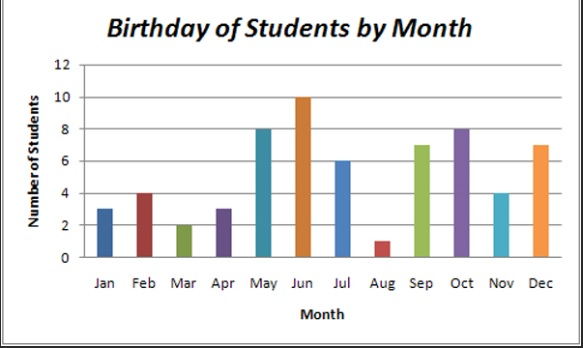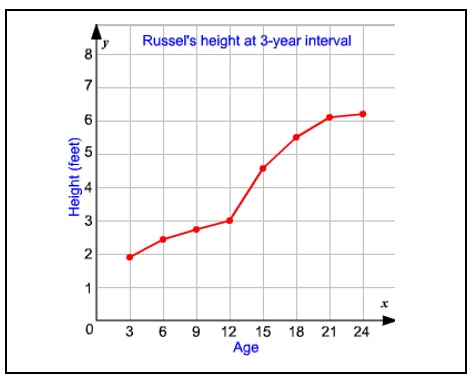Charts and Graphs

Many people find it easier to understand quantitative data when it is presented in pictorial form. The specification requires that you  look at 3 types of pictorial presentations of data.

1) BAR CHARTS

A simple and effective way of presenting and comparing data, particularly nominal data. This is because each bar represents a different category of data, and this is denoted by the spaces between them (it is important to leave a gap/space betweeneach bar on the graph in order to indicate that the bars represent ‘separate’ data rather than ‘continuous’ data. Different categories of data are known as discrete data. The bars can be drawn in any order.

Graph example taken from Wiki Educator.org

2) HISTOGRAMS

These are mainly used to present frequency distributions of interval data. The horizontal axis is a continuous scale, (e.g. time in seconds). On a histogram, there are no spaces between bars, because the bars are not considered separate categories. In the example of the graph to the right, the data shows how many students have achieved between a score of 20-30, 30-40, 40-50 etc… marks on a test. The data is interval (i.e. between 20-30 marks) and is continuous.

3) LINE GRAPH/LINE CHART

A line chart or line graph is a type of chart which displays information as a series of data points called ‘markers‘ connected by straight line segments. It is similar to a scatter graph except that the measurement points are ordered and joined with straight line segments (showing that the data is continuous).